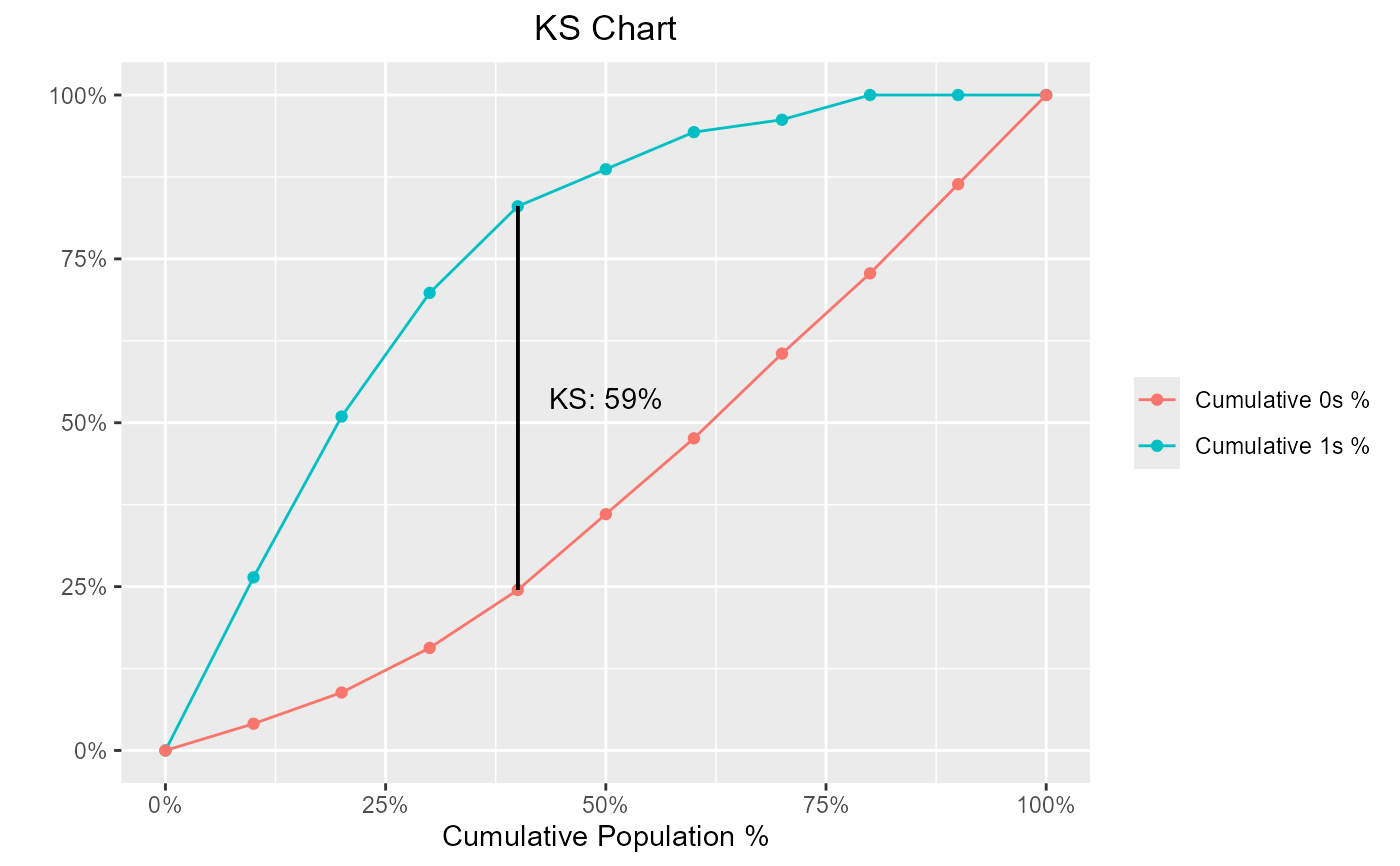Kolmogorov-Smirnov (KS) statistics is used to assess predictive power for marketing or credit risk models. It is the maximum difference between cumulative event and non-event distribution across score/probability bands. The gains table typically has across score bands and can be used to find the KS for a model.

blr_ks_chart(
gains_table,
title = "KS Chart",
yaxis_title = " ",
xaxis_title = "Cumulative Population %",
ks_line_color = "black",
print_plot = TRUE
)

## Arguments

gains_table An object of class blr_gains_table. Plot title. Y axis title. X axis title. Color of the line indicating maximum KS statistic. logical; if TRUE, prints the plot else returns a plot object.

https://doi.org/10.1198/tast.2009.08210

https://www.ncbi.nlm.nih.gov/pubmed/843576

## See also

Other model validation techniques: blr_confusion_matrix(), blr_decile_capture_rate(), blr_decile_lift_chart(), blr_gains_table(), blr_gini_index(), blr_lorenz_curve(), blr_roc_curve(), blr_test_hosmer_lemeshow()

## Examples

model <- glm(honcomp ~ female + read + science, data = hsb2,
family = binomial(link = 'logit'))
gt <- blr_gains_table(model)
blr_ks_chart(gt)